# Multimap in C++ STL

C++ STL | Multimap: In this tutorial, we are going to learn about the multimap, what is multimap in C++ STL along with its application and use cases?
Submitted by Radib Kar, on June 18, 2020

## multimap in C++

C++ STL is an enriched functionality that is similar to Java Collection providing us the black-box of built-in data structures to use in our codes. It saves 100 lines to write and STL is a popular feature and advantage of C++ widely used in competitive programming.

Those who are familiar with C++ STL must have used the STL map and unordered_map as part of their coding usage.

Multimap is an extended version of the map which can be very useful in many cases. Let's dig into the comparison b/w map and multimap to get proper understanding.

Say we have 5 keys with corresponding values like below,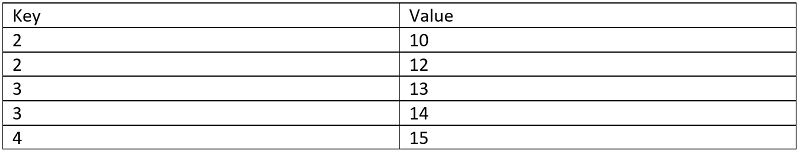Let's use STL map to store the above.

After inserting first <key, value> pair the map will be,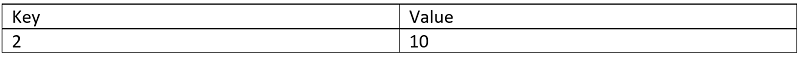Now when we will insert the next <key, value> pair, we can see that the key already exists, so it will not make any new entry. It will simply update the key value in the map.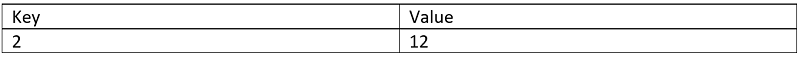So, after similar insertion the final map will be,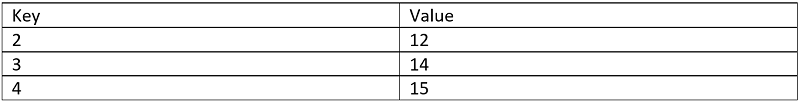But what if we want all the entries persisting then what to do. Simply, maps will not work there. That's why we have a multimap in CPP STL. In multimap, we can have different entries based on even the same key. So, keys with different values will have a separate entry in multimap and that’s the power of multimap.

So, whenever you need to store each distinct <key, value> pair we can use Multimap in such cases.

So after entering the above entries the final multimap will be like,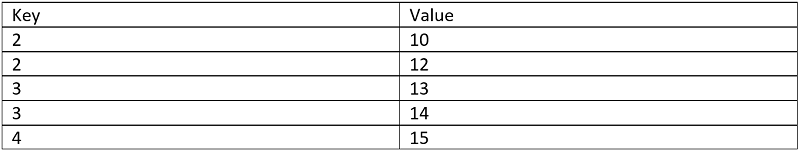Multimap also stores the keys in sorted order and has the same time complexity as the map.

To declare a multimap,

```multiplate <int,int> mymap;
```

Below are the functions available on multimap,

Function Description
erase() It is used to erase the elements.
insert() It is used to insert elements.
begin() It is used to get an iterator to beginning.
end() It is used to get an iterator to end.
empty() It is used to check whether the multimap is empty or not.
size() It is used to get the size of the multimap.
maxsize() It is used to get the maximum size.
lower_bound() It is used to get the iterator to the lower bound.
upper_bound() It is used to get the iterator to the upper bound.

## C++ implementation of multimap

```#include <bits/stdc++.h>
using namespace std;

int main()
{
multimap<int, int> mymultimap;
map<int, int> mymap;

//insertion in map
mymap = 10;
mymap = 12;
mymap = 13;
mymap = 14;
mymap = 15;

//insertion in multimap
mymultimap.insert(make_pair(2, 10));
mymultimap.insert(make_pair(2, 12));
mymultimap.insert(make_pair(3, 13));
mymultimap.insert(make_pair(3, 14));
mymultimap.insert(make_pair(4, 15));

cout << "Printing the map\n";

for (auto it = mymap.begin(); it != mymap.end(); it++) {
cout << "key: " << it->first << ", value: " << it->second << endl;
}

cout << "Printing the multimap\n";

for (auto ij = mymultimap.begin(); ij != mymultimap.end(); ij++) {
cout << "key: " << ij->first << ", value: " << ij->second << endl;
}

return 0;
}
```

Output:

```Printing the map
key: 2, value: 12
key: 3, value: 14
key: 4, value: 15
Printing the multimap
key: 2, value: 10
key: 2, value: 12
key: 3, value: 13
key: 3, value: 14
key: 4, value: 15
```

Preparation